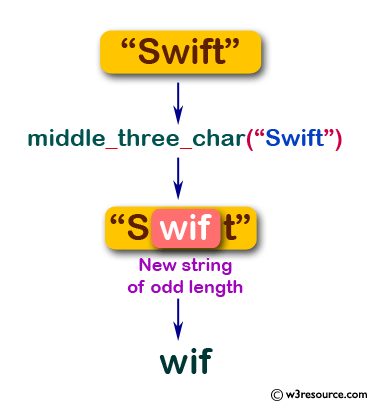﻿ Swift String Programming Exercise: Create a new string of length three from a given string of odd length from its middle - w3resource# Swift String Exercises: Create a new string of length three from a given string of odd length from its middle

## Swift String Programming: Exercise-15 with Solution

Write a Swift program to create a new string of length three from a given string of odd length from its middle. The string length must be three.

Pictorial Presentation:Sample Solution:

Swift Code:

``````import Foundation
func middle_three_char(_ input: String) -> String {
let chars = input.characters
let middle_num = (chars.count - 1) / 2
let first_num = middle_num - 1
let third_num = middle_num + 1

let first_index = input.index(chars.startIndex, offsetBy: first_num)
let third_index = input.index(chars.startIndex, offsetBy: third_num + 1)
let middle_range = first_index ..< third_index
let result = input.substring(with: middle_range)

return result
}
print(middle_three_char("Swift"))
print(middle_three_char("abcde"))
```
```

Sample Output:

```
wif
bcd
```

Swift Programming Code Editor:

Improve this sample solution and post your code through Disqus

What is the difficulty level of this exercise?

﻿